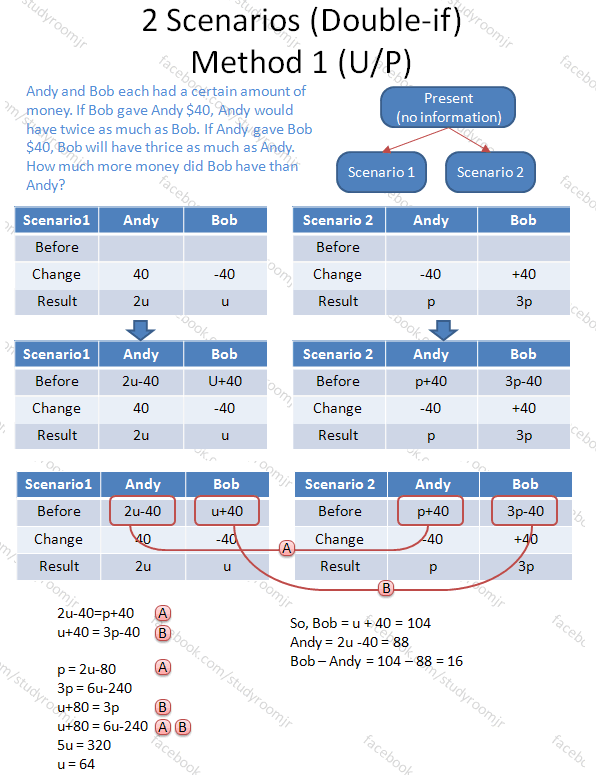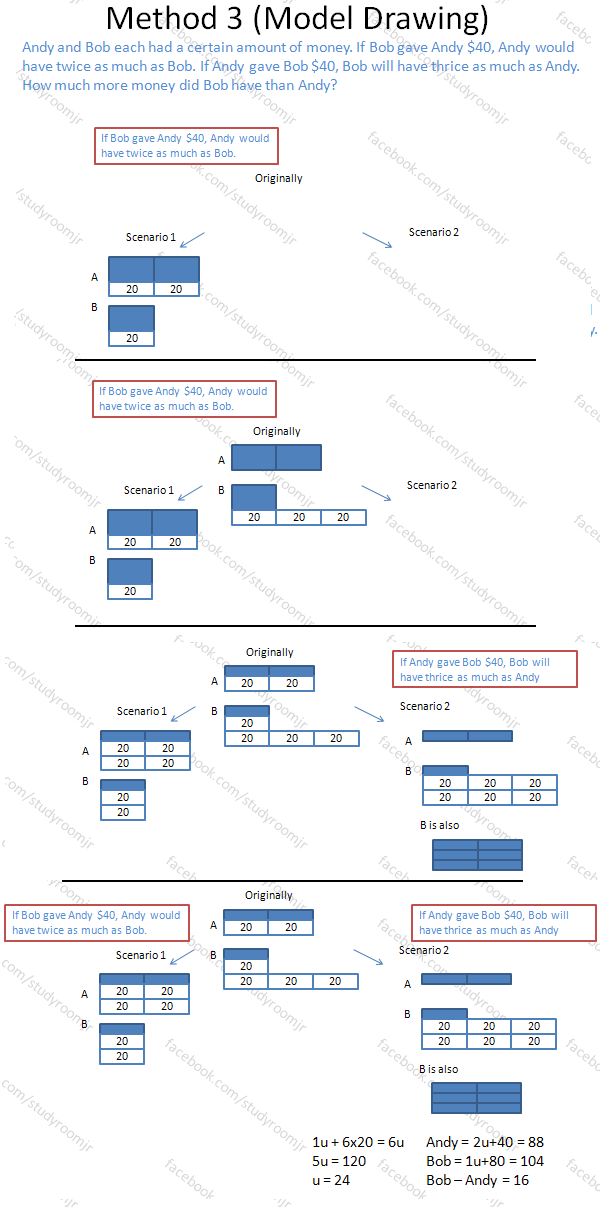# Double-if questions – Methods of solvingMaths tip:

Scenario type questions, sometimes also called "Double-if" questions, are common in the last section of the Maths paper. An example is "Andy and Bob each had a certain amount of money. If Bob gave Andy \$40, Andy would have twice as much as Bob. If Andy gave Bob \$40, Bob will have thrice as much as Andy. How much more money did Bob have than Andy?"

Here is the first of three different methods you can use to solve these types of problems.

This is also known as the "unit / parts" method or "UP" method. It is similar to algebra, and can be used to solved many other questions as well.

Note that before both scenarios, Andy and Bob have the same amount of money. This can be equated to each other.

Maths tip:

Here’s method 2 for "double-if" questions. This is a "cross-multiply" method.

The idea behind it is simple. If the weight of a watermelon is five times the weight of an apple, we write the ratio as 5:1.
i.e. weight of watermelon : weight of apple = 5 : 1
this also means that weight of 5 apples = weight of 1 water melon.

Thus, to solve with this method, first determine the "before" in scenario 1, add the change in scenario 2, and cross multiply with the final ratio!

Here is Method 3 for "double-if" type questions. This is the model drawing method. The entire drafting process is illustrated.

This method is the most visual. However, the model can vary quite a bit between the various questions (even among questions of the same type) so it requires more thinking and understanding than the previous two methods. Nonetheless, for students more comfortable with model drawing, it is a viable method.